# How to use the AVEDEV function in Excel

The average deviation of a set of values is calculated by taking the mean and then specific difference between each score and that mean without regard to whether the score is above or below the mean. It is also called as average absolute deviation. Below mentioned is the formula to calculate the average deviation.

AVEDEV function returns a number which is the Average deviation of the set of data in Excel.
Syntax:

=AVEDEV(number1, [number2],...)

Let’s understand this function using it in an example.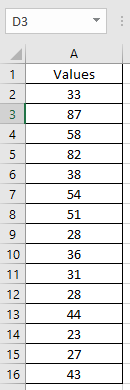Here we have a list of values and we need to get the Average deviation from the mean of numbers.
Use the formula:

=AVEDEV(A2:A16)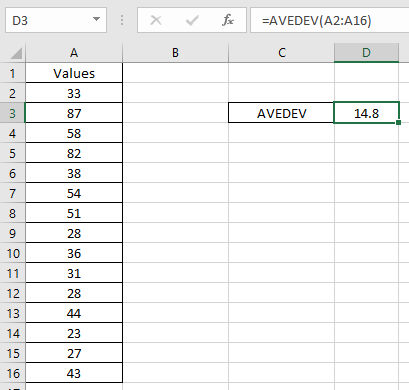As you can see the formula returns 14.8.
This must be confusing but the below explanation will make you understand better.
Take the average of the values.
Use the formula:

=AVERAGE(A2:A16)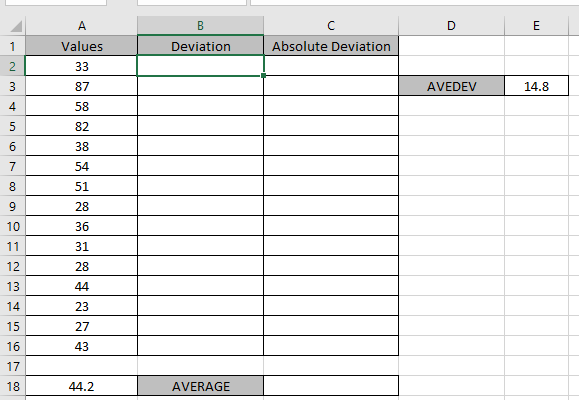As you can see, we got the average of the numbers, in the snapshot above.
Now we will take the deviation of the numbers from the average value. Deviation of a number from the array is calculated by taking its difference from the AVERAGE of array.

= \$A\$18 - A2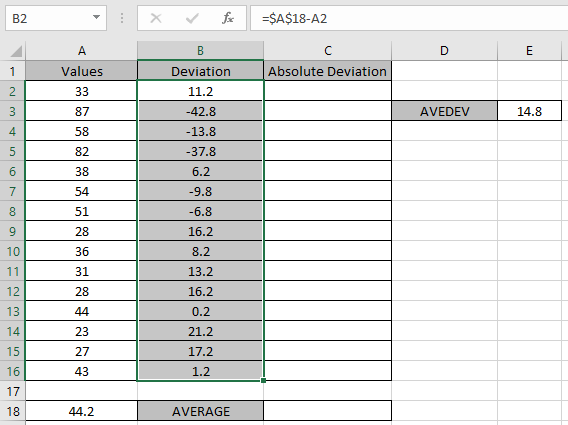Now take the absolute value of the deviation values.
Use the formula:

=ABS(B2)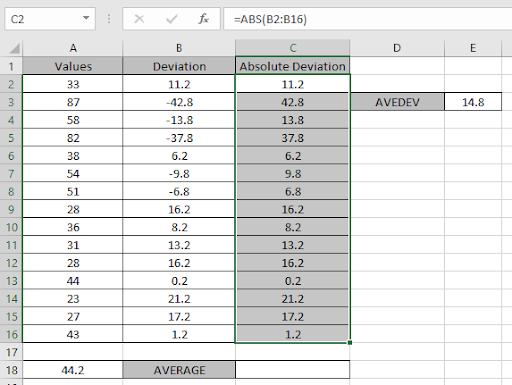Take the average of the values.
Use the formula:

=AVERAGE(C2:C16)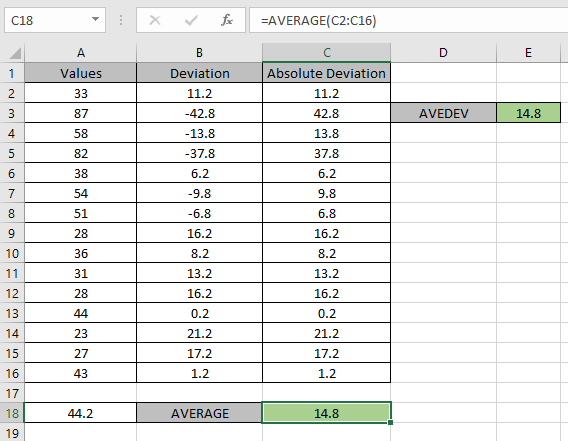This is the explanation for the function AVEDEV function in Excel.

I hope the article about How to use the AVEDEV function in Excel was helpful. Explore more articles on Statistical functions here. Please state your query or feedback in the comment box below.

Related Data:
How to Use STDEV Function in Excel

How to Use STDEV.S Function in Excel

Regressions Analysis in Excel

How To Calculate MODE

How To Calculate Mean

How to Create a Standard Deviation Graph

Popular Articles:

50 Excel Shortcuts to Increase Your Productivity

How to use the VLOOKUP Function in Excel

How to use the COUNTIF in Excel 2016

How to use the SUMIF Function in Excel

Terms and Conditions of use

The applications/code on this site are distributed as is and without warranties or liability. In no event shall the owner of the copyrights, or the authors of the applications/code be liable for any loss of profit, any problems or any damage resulting from the use or evaluation of the applications/code.